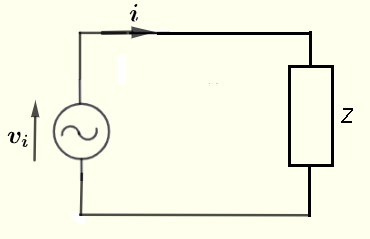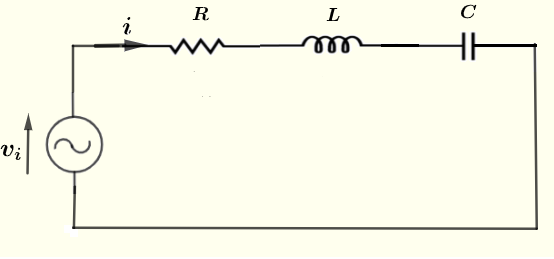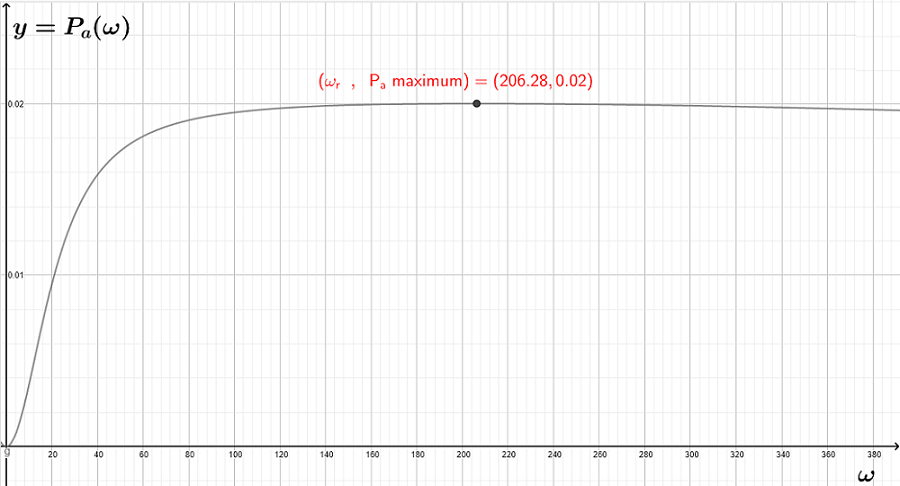# Power in AC Circuits

   

The calculation of the average power in ac circuits is presented with examples and their solutions. Problems with solutions are also included.

## A - Instantaneous Power in AC Circuits

Consider the circuit belowLet the impedance $Z$ be written in polar form as $Z = |Z| \; e^{j\theta}$
Let $v_i (t) = V_0 \; \cos(\omega t)$
hence
$i (t) = \dfrac{V_0}{|Z|} \; \cos (\omega t - \theta)$
The instantenaous power $P(t)$ delivered to the impedance $Z$ is given by
$P(t) = i(t) \; v(t) = \dfrac{V_0^2}{|Z|} \; \cos ( \omega t - \theta) \; \cos(\omega t)$

## B - Average Power in AC Circuits

The average power is defined by
$P_a = \displaystyle \dfrac{1}{T} \int_0^T P(t) dt$
Substitute $P(t)$ by the expression found above and write the average power as
$P_a = \displaystyle \dfrac{V_0^2}{T |Z|} \int_0^T \; \cos ( \omega t - \theta) \; \cos(\omega t) \; dt$
Expand: $\quad \cos ( \omega t - \theta) = \cos \omega t \; \cos \theta + \sin \omega t \; \sin \theta$ and substitute in $P_a$
$P_a = \displaystyle \dfrac{V_0^2}{T |Z|} \int_0^T \; (\cos^2 \omega t \; \cos \theta + \sin \omega t \; \cos \omega t \; \sin \theta ) \; dt$
Write the integral as a sum of two integrals: one integral on the left and a second integral on the right as follows:
$P_a = \displaystyle \dfrac{V_0^2}{T |Z|} \int_0^T \; cos^2 \omega t \; \cos \theta \; dt + \dfrac{V_0^2}{T |Z|} \int_0^T \; \sin \omega t \; \cos \omega t \; \sin \theta \; dt$
Using the trigonometric identity: $\quad \sin(\omega t) \cos(\omega t) = \dfrac{1}{2} \sin(2 \omega t)$ to rewrite the integral on the right as
$\displaystyle \dfrac{V_0^2}{T |Z|} \int_0^T \; \sin \omega t \; \cos \omega t \; \sin \theta \; dt = \dfrac{V_0^2}{2 T |Z|} \sin \theta) \int_0^T \sin (2 \omega t ) \; dt$
$\quad = - \displaystyle \dfrac{V_0^2}{2 T |Z|} \sin \theta \dfrac{1}{2 \omega } \left[\cos (2 \omega t ) \right]_0^T$
$\quad \quad = - \displaystyle \dfrac{V_0^2}{2 T |Z|} \sin \theta \dfrac{1}{2 \omega } \left[\cos 2 \omega T - \cos 0 \right]$
Use the formula $\quad \omega = \dfrac{2 \pi}{T}$ to simplify $\cos 2 \omega T$
$\quad \quad \quad = - \displaystyle \dfrac{V_0^2}{2 T |Z|} \sin \theta \dfrac{1}{2 \omega } [\cos (4 \pi) - \cos 0]$
$\quad \quad \quad \quad = 0$

Use the trigonometric identity $\quad \cos^2 \omega t = \dfrac{1}{2} (\cos(2 \omega t )+1)$ in the integral on the left and write
$P_a = \displaystyle \dfrac{V_0^2}{2 T |Z|} \; \cos \theta \; \int_0^T \; (\cos(2 \omega t )+1) \; dt$
Write the integral as a sum of two integrals
$P_a = \displaystyle \dfrac{V_0^2}{2 T |Z|} \cos \theta \int_0^T \; \dfrac{1}{2} \cos(2 \omega t ) \; dt + \dfrac{V_0^2}{2 T |Z|} \; \cos \theta \; \int_0^T \; dt$
In a similar way as above, it can be shown that $\displaystyle \int_0^T \; \dfrac{1}{2} \cos(2 \omega t ) \; dt = 0$
Hence $P_a$ is given by
$\displaystyle P_a = \dfrac{V_0^2}{2 T |Z|} \; \cos \theta \; \int_0^T \; dt$
$\displaystyle \quad = \dfrac{V_0^2}{2 T |Z|} \; \cos \theta \left[t\right]_0^T$
$\displaystyle \quad \quad P_a = \dfrac{V_0^2}{2 |Z|} \cos \theta$
The term $\cos \theta$ in the formula above is called the power factor.
Note that, in general, $|Z|$ and $\cos \theta$ depend on the frequency and therefore the average power depend on the frequency of the voltage (or current) source.
As shown above the calculations could be quite challenging and therefore a power calculator for series RLC circuit is included for more practice and investigations.

Example 1
In the series RLC circuit shown below, the source voltage is given by $v_i = 5 \cos (\omega t)$, the capacitance of the capacitor $C = 100 \; \mu F$, the inductance of the inductor $L = 100 \; mH$ and the resistance of the resistor $R = 1000 \; \Omega$ and frequency $f = 2000 \; Hertz$.a) Find the total impedance $Z$ of the series RLC circuit and express it in polar form.
b) Find the average power delivered to the total impedance $Z$.

Solution to Example 1
a)
For a series RLC circuit $Z = R + j(\omega L - \dfrac{1}{\omega C} )$
$\omega = 2 \pi f = 4000 \pi$ rad/s
Substitute $R$, $L$, $C$ and $\omega$ by their numerical values to obtain
$Z = 1000 + j\left(4000 \pi \times 100 \times 10^{-3} - \dfrac{1}{4000 \pi \times 100 \times 10^{-6}} \right)$
$Z = 1000 + \left(400\pi -\dfrac{5}{2\pi} \right) j$
The impedance $Z$ is written in standard complex form $Z = a + j b$
In polar form the same impedance is written as $Z = |Z| e^{j\theta}$
where $\theta = \arctan \dfrac{b}{a}$ and $|Z| = \sqrt {a^2 + b^2}$
Hence
$\theta = \arctan \left(\dfrac{400\pi -\dfrac{5}{2\pi} }{1000} \right)$
$|Z| = \sqrt {1000^2 + \left(400\pi -\dfrac{5}{2\pi}\right)^2}$

b)
$\displaystyle P_a = \dfrac{V_0^2}{2 |Z|} \cos \theta$
Substitute $V_0$, $|Z|$ and $\theta$ by their numerical values
$\displaystyle P_a = \dfrac{5^2}{2 \sqrt {1000^2 + \left(400\pi -\dfrac{5}{2\pi}\right)^2} } \cos \left( \arctan \left(\dfrac{400\pi -\dfrac{5}{2\pi} }{1000} \right) \right)$
$\quad \approx 0.00485 \; \text{Watts}$

Example 2
Show that the average power delivered to a series RLC circuit, such as the one in example 1, is maximum for a frequency $f = \dfrac{1}{2 \pi \sqrt{LC}}$ and find a formula for this maximum power.

Solution to Example 2
a) For a series RLC circuit, the total impedance is given by:   $Z = R + j(\omega L - \dfrac{1}{\omega C})$
The angular frequency $\omega$ is related to the frequency $f$ by the formula: $\omega = 2 \pi f = \dfrac{1}{\sqrt{LC}}$
Substitute $\omega$ by $\dfrac{1}{\sqrt{LC}}$ in $Z$ to obtain
$Z = R + j (\dfrac{1}{\sqrt{LC}} L - \dfrac{1}{\dfrac{C}{\sqrt{LC}}})$
$\quad = R + j ( \dfrac{1}{\sqrt{LC}} L - \dfrac{\sqrt{LC}}{C} )$
$\quad = R + j ( \dfrac{\sqrt L}{\sqrt C} - \dfrac{\sqrt{L}}{\sqrt C} )$
which simplifies to
$Z = R$
For the frequency $f = \dfrac{1}{2 \pi \sqrt{LC}}$, the impedance $Z$ is real and therefore
$|Z| = R$
and the argument $\theta$ of $Z$ is equal to zero. Hence $\cos \theta = \cos 0 = 1$ has a maximum value.
For the frequency $f = \dfrac{1}{2 \pi \sqrt{LC}}$, the power factor $\cos \theta = \cos 0 = 1$ is maximum and $|Z|$ is minimum which gives an average power with a maximum value given by
$P_a max = \dfrac{V_0^2}{2 R}$

Example 3
In a series RLC circuit, such as the one in example 1 above, the source voltage is given by $v_i = 2 \cos ( \omega t)$, the capacitance of the capacitor $C = 470 \mu$F, the inductance of the inductor $L = 50$mH and the resistance of the resistor is $R = 100 \; \Omega$.
a) Express the average power $P_a$ given by its formula above and make a graph of $P_a$ as a function of the angular frequency $\omega$ and find the location of the maximum of $P_a$.
b) Check that the power is maximum at the angular $\omega_r = \dfrac{1}{\sqrt{LC}}$ (or the frequency $f_r = \dfrac{1}{2 \pi \sqrt{LC}}$) and is given by $P_a max = \dfrac{V_0^2}{2 R}$ as explained in example 2 above.

Solution to Example 3
For a series RLC circuit $Z = R + j(\omega L - \dfrac{1}{\omega C})$
Modulus: $|Z| = \sqrt { R^2 + \left( \omega L - \dfrac{1}{\omega C} \right)^2}$

Argument: $\theta = \arctan \left( \dfrac{\omega L - \dfrac{1}{\omega C}} {R} \right)$
The average power $P_a$ is given by
$P_a = \dfrac{V_0^2}{2 |Z|} \cos \theta$
Substitute $V_0$ and $|Z|$ by their expressions
$P_a (\omega ) = \dfrac{V_0^2}{2 \sqrt { R^2 + \left( \omega L - \dfrac{1}{\omega C} \right)^2}} \cos \left(\arctan \left( \dfrac{\omega L - \dfrac{1}{\omega C}} {R} \right) \right)$
Substitute $R$, $L$ and $C$ by their values to obtain $P_a$ as a function of $\omega$
$P_a (\omega ) = \dfrac{2}{ \sqrt { 100^2 + \left( 50 \times 10^{-3} \; \omega - \dfrac{1}{470 \times 10^{-6}\; \omega } \right)^2}} \cos \left(\arctan \left( \dfrac{50 \times 10^{-3} \; \omega - \dfrac{1}{ 470 \times 10^{-6} \; \omega }} {100} \right) \right)$
The graph of $P_a (\omega )$ against $\omega$ is shown below.
The free geogebra graphing calculator was used to graph and locate the maximum as shown in the graph.b)
In example 2 above, it was explained the average power is maximum at
$\omega_r = \dfrac{1}{\sqrt{LC}} = \dfrac{1}{ \sqrt{50 \times 10^{-3} \times 470 \times 10^{-6} }} \approx 206.28$ rad/s
The maximum power is given by: $P_a max = \dfrac{V_0^2}{2 R} = \dfrac{2^2}{2 \times 100} = 0.02$ Watts
Both the calculated values of $\omega_r$ and $P_a max$ calculated are equal to values found graphically above.